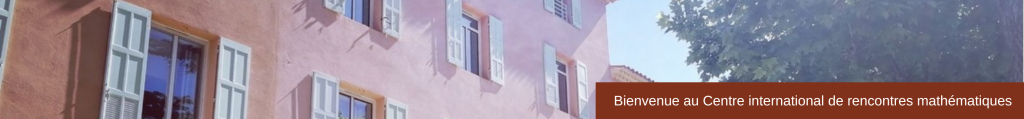RESEARCH IN PAIRS

Complete Kahler-Einstein Metrics and Gravitational Instantons
June 1 – 19, 2015
 The proposed research for the three-week ”Research in Pairs”  with Prof. Ioana  Suvaina  (Vanderbilt University),  is part  of a longer project  aimed at classifying all Ricci-flat Kähler metrics on complex surfaces. In the Trieste  Summer School of 2008, Tian made  a conjecture that  it is possible to completely classify all complete Ricci-flat Kähler metrics on surfaces  in terms  of the  volume  growth  of the  metric:  ALE  (Euclidean), ALF (cubic), ALG, or ALH. Suvaina, in [Su], provides a complete and explicit classification of the ALE Ricci-flat Kähler surfaces. This is a generalization  of Kronheimer’s results on hyper Kähler manifolds [K], and establishes the correspondence between ALE Ricci-flat Kähler manifolds and a special class of deformations, Q-Gorenstein, of isolated quotient singularities. Rasdeaconu-Suvaina, in [RS], analyzes the relation between the construc- tion in [Su] and  the  metrics constructed by Tian and  Yau [TY1]  [TY2] on the complement of a divisor in a compact surface. These two  results  complete  the  proof of Tian’s conjecture for the  ALE case, and provide the relations between all know examples. During our three-week stay at CIRM, we now propose to attack  the case of ALF metrics:  relating  the Ricci-flat Kähler metrics  constructed by Tian and Yau on the complement of a divisor on a compact surface, to the explicit examples  of Asymptotically  Locally Flat  (ALF)  metrics:   the  multi-Taub- NUT metrics of Hawking [H], LeBrun [L], Cherkis-Hitchin  [CH]. In the case of ALF hyper Kähler manifolds of cyclic type, Minerbe [M] completed the classification showing that  the only possible examples are the trivial R3 × S1, or the multi-Taub-NUT metrics.   Such a classification is unknown  if one  considers  the  more  general  case  of ALF  Rici-flat  Kähler surfaces (not necessary hyper kähler), or if the topological structure at infinity is not specified.   For  example,  in [Su] examples  of ALF  Ricci-flat Kähler surfaces with cyclic fundamental  group at  infinity are presented.  They are not of cyclic type, and are obtained as quotients of the hyper kähler cases. In Biquard-Minerbe  [BM], a uniform approach, which considers minimal resolutions of quotients of gravitational instantons is presented, and they recover a member of nearly  all deformation classes of known Ricci-flat hy- perk kähler surfaces with ALF, ALG and ALH asymptotics. Our project  will focus on relating  the construction  in [BM] to the solutions of the complex Monge-Ampère equation given by [TY1] and [TY2]. On the lines of [S1], which describe the asymptotic behavior of the Tian-Yau metrics, and of [S2], which solves the complex Monge-Ampère equation with prescribed asymptotics, we plan to study conditions for uniqueness of solu- tions  to  the  Monge-Ampère equation, and  to use such results to partially classify the  remaining  Ricci-flat Kähler cases. In particular, we expect  to obtain  ALF Ricci-flat Kähler metrics on the whole family of deformations of the B iquard-Minerbe examples, as well as their quotients. ParticipantsBianca Santoro (City University of New York)Ioana Suvaina (Vanderbilt University) Sponsor